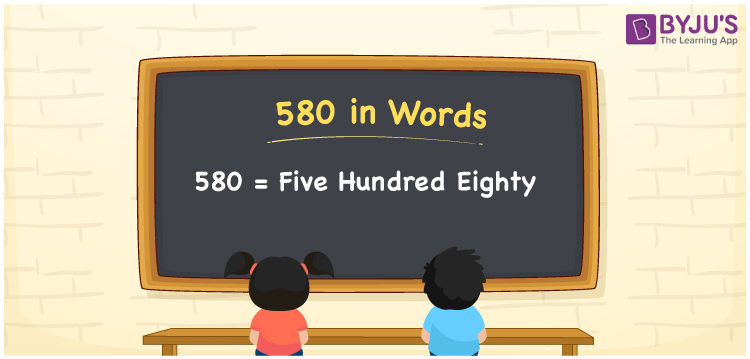# 580 in Words

The number 580 in words is “Five Hundred Eighty. In both the International and the Indian System of Numerals, the number 580 in words is written as “Five Hundred Eighty”. The number 580 is a cardinal number as it represents the value or the quantity of the objects. For example, “There are 580 apples in a fruit shop”. This can be written as “There are five hundred eighty apples in a fruit shop”.

 580 in Words: Five Hundred Eighty. Five Hundred Eighty in Numerical Form: 580.

## 580 in English Words

In English words, the number 580 is spelt as “Five Hundred Eighty”.## How to Write 580 in Words?

We will use the place value chart to write the number 580 in words. To write the number 580 in English, let’s create a place value chart.

 Hundreds Tens Ones 5 8 0

The expanded form of 580 is as follows:

= 5 × Hundred + 8 × Ten + 0 × One

= 5 × 100 + 8 × 10

= 500 + 80

= 580

= Five hundred eighty

Hence, 580 in words is Five hundred eighty.

580 is a natural number, which is the successor of 579 and the predecessor of 581.

580 in words – Five hundred eighty

Is 580 an odd number? – No

Is 580 an even number? – Yes

Is 580 a perfect square number? – No

Is 580 a perfect cube number? – No

Is 580 a prime number? – No

Is 580 a composite number? – Yes

## Frequently Asked Questions on 580 in Words

Q1

### Write 580 in words.

580 in words is five hundred eighty.

Q2

### Simplify 500 + 80, and express in words.

Simplifying 500 + 80, we get 580. Hence, 580 in words is five hundred eighty.

Q3

### Is 580 a composite number?

Yes, 580 is a composite number.